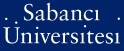# On isomorphisms of spaces of analytic functions of several complex variables

Karıksız, Can Deha (2007) On isomorphisms of spaces of analytic functions of several complex variables. [Thesis]Preview
PDF - Requires a PDF viewer such as GSview, Xpdf or Adobe Acrobat Reader
202Kb

## Abstract

In this thesis, we discuss results on isomorphisms of spaces of analytic functions of several complex variables in terms of pluripotential theoretic considerations. More specifically, we present the following result: Theorem 1 Let Ω be a Stein manifold of dimension n. Then, A(Ω) ≈ A(U[n]) if and only if Ω is pluriregular and consists of at most finite number of connected components. The problem of isomorphic classification of spaces of analytic functions is also closely related to the problem of existence and construction of bases in such spaces. The essential tools we use in our approach are Hilbert methods and the interpolation properties of spaces of analytic functions which give us estimates of dual norms and help us to obtain extendable bases for pluriregular pairs.

Item Type: Thesis Interpolation. -- Duality. -- Pluripotential theory. -- Stein manifolds. -- Spaces of analytic functions. -- Pluriregularity. Q Science > QA Mathematics 11347 IC-Cataloging 25 Feb 2009 17:16 25 Mar 2019 16:57

Repository Staff Only: item control page